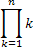A | B | C | D | E | F | G | H | I | J | K | L | M | N | O | P | Q | R | S | T | U | V | W | X | Y | Z

# Mathematics-Factorial

Factorial of a non-negative integer, n is denoted as n!. And it is calculated using product of all positive integers less than or equal to n.

Eg ) 5! = 5 * 4 * 3 * 2 * 1 = 120

The notation n! is introduced by Christian Kramb.

Factorial function defined normally by  n! =or it is denoted recursively by n! =   1                 if n = 0
(n - 1)! * n    if n > 0

Factorial function is denoted by using a symbol  ' ! ' . It means to multiply a series of descending natural numbers.
4! = 4 * 3 * 2 * 1 = 24

Main usage of factorial is in permutation & combination

Half Factorial ::

Factorial of a half ( 1/2 ) is half of the square root of ( pi ) ∏.
eg ) ( 1/2 ) ! = ( 1/2 )√

To conclude factorial of a any number is that number times the factorial of 1 less than that number.